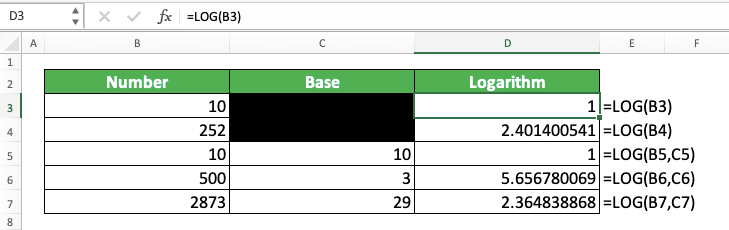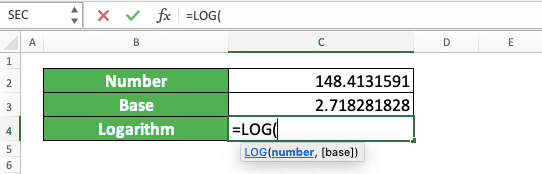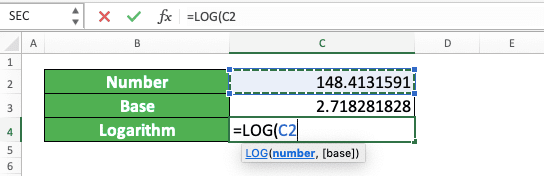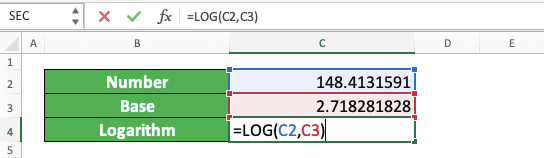How to Use the LOG Function in Excel: Usabilities, Examples, and Writing Steps - Compute Expert

# How to Use the LOG Function in Excel: Usabilities, Examples, and Writing Steps

Home >> Excel Tutorials from Compute Expert >> Excel Formulas List >> How to Use the LOG Function in Excel: Usabilities, Examples, and Writing Steps

In this tutorial, you will learn how to use the LOG function in excel completely.

When working with numbers in excel, we might sometimes need to calculate the logarithm of our number using a certain base. If we know how to use the LOG function in excel, then we can get our number logarithm easily.

Want to know more about LOG and master the way to use this function in excel? Read this tutorial until its last part!

Disclaimer: This post may contain affiliate links from which we earn commission from qualifying purchases/actions at no additional cost for you. Learn more

## What is the LOG Function in Excel?

LOG is an excel function that helps you to calculate the logarithm of a number in excel fast and easily.

## LOG Usability

You can use LOG to get the logarithm of a number.

## LOG Result

The LOG result is a number that represents the logarithm of a number you input into it.

## The Way to Write It and Its Input

= LOG ( number , [ base ] )

And here is a bit explanation of the inputs you need to give when you use LOG in excel.
• number = the number you want to find the logarithm of
• [base] = optional. The base for your logarithm calculation. If you omit this, then LOG will calculate the logarithm of your number on the base of 10

## Example of Its Usage and InputIn the example, you can see how LOG can help us to get the logarithm of our number. Just input the number we want to get the logarithm of and the logarithm base if needed and press Enter. You will immediately get your logarithm result by doing that!

## Writing Steps

1. Type an equal sign ( = ) in the cell where you want to put the logarithm of your number2. Type LOG (can be with large and small letters) and an open bracket sign after =3. Input the number you want to get the logarithm of4. Optional: Type a comma sign. Then, input the base you want for your logarithm calculation. If you don’t give any base input here, LOG will calculate the logarithm of your number on the base of 105. Type a close bracket sign6. Press Enter
7. Done!## Exercise

### Instructions

Use LOG to answer all the questions below! Give your answer for each question in the appropriate gray-colored cell!
1. Find the logarithm of the number in the no.1 row with the logarithm base of 10!
2. Find the logarithm of the number in the no.2 row with the logarithm base of 2.5!
3. Find the natural logarithm of the number in the no.3 row! (Hint: the natural logarithm is a logarithm with the base of an e constant or Euler’s number. You can use the EXP(1) formula writing to get that number for you in excel!)

You can only input a positive number to LOG. Other kinds of input will make LOG gives you an error.

Related tutorials you should learn:

Get updated excel info from Compute Expert by registering your email. It's free!